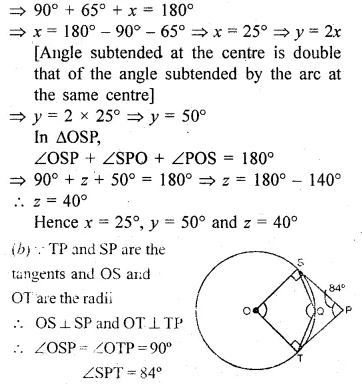Guru

# Question 22. (a) In the figure (i) given below, O is the centre of the circle and SP is a tangent. If ∠SRT = 65°, find the value of x, y and z. (2015) (b) In the figure (ii) given below, O is the centre of the circle. PS and PT are tangents and ∠SPT = 84°. Calculate the sizes of the angles TOS and TQS.

• -1

This is circle based question from Chapter name- circles
Topic – Angle properties of circles
Chapter number- 15

In this question we have, O is the centre of the circle and SP is a tangent.

∠SRT = 65°, and by using these we have to find the value of x, y and z.

This question has been asked in 2015.

In the second part of the question we have O is the centre of the circle. PS and PT are tangents and ∠SPT = 84°.

Now we have to calculate the sizes of the angles TOS and TQS.

ICSE Avichal publication
Understanding ICSE Mathematics
Question no 22

Share